Area between Curves

Now, when we know what definite integral is, we can calculate area between curves.

Consider the region ${S}$ that lies between two curves ${y}={f{{\left({x}\right)}}}$ and ${y}={g{{\left({x}\right)}}}$ and between the vertical lines ${x}={a}$ and ${x}={b}$, where ${f{}}$ and ${g{}}$ are continuous functions and ${f{{\left({x}\right)}}}\ge{g{{\left({x}\right)}}}$ for all ${x}$ in ${\left[{a},{b}\right]}$.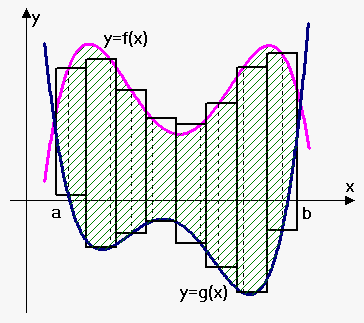We will proceed in the same fashion as we did when talked about area problem.

Divide region into ${n}$ strips of wdith $\Delta{x}=\frac{{{b}-{a}}}{{n}}$. We approximate i-th strip by rectangle with base $\Delta{x}$ and height ${f{{\left({{x}_{{i}}^{{\star}}}\right)}}}-{g{{\left({{x}_{{i}}^{{\star}}}\right)}}}$ where ${{x}_{{i}}^{{\star}}}\in{\left[{x}_{{{i}-{1}}},{x}_{{i}}\right]}$. Thus, approximate area is defined by Riemann sum as ${A}\approx{\sum_{{{i}={1}}}^{{n}}}{\left({f{{\left({{x}_{{i}}^{{\star}}}\right)}}}-{g{{\left({{x}_{{i}}^{{\star}}}\right)}}}\right)}\Delta{x}$. As ${n}$ becomes larger, approximation becomes better.

Therefore, we define area A of S as the limiting value of the sum of the areas of these approximating rectangles: ${A}=\lim_{{{n}\to\infty}}{\sum_{{{i}={1}}}^{{n}}}{\left({f{{\left({{x}_{{i}}^{{\star}}}\right)}}}-{g{{\left({{x}_{{i}}^{{\star}}}\right)}}}\right)}\Delta{x}$.

We recognize in limit definite integral of ${f{-}}{g{}}$.

Formula for Area between Curves. The area ${A}$ of the region bounded by the curves ${y}={f{{\left({x}\right)}}}$ and ${y}={g{{\left({x}\right)}}}$, and the lines ${x}={a}$, ${x}={b}$, where ${f{}}$ and ${g{}}$ are continuous functions and ${f{{\left({x}\right)}}}\ge{g{{\left({x}\right)}}}$ for all ${x}$ in ${\left[{a},{b}\right]}$ is ${A}={\int_{{a}}^{{b}}}{\left({f{{\left({x}\right)}}}-{g{{\left({x}\right)}}}\right)}{d}{x}$.

Indeed, we know that if ${f{}}$ is positive then area under function ${f{{\left({x}\right)}}}$ and above x-axis on ${\left[{a},{b}\right]}$ is ${\int_{{a}}^{{b}}}{f{{\left({x}\right)}}}{d}{x}$. In case ${f{{\left({x}\right)}}}$ is negative, area above function ${f{{\left({x}\right)}}}$ and below x-axis is again ${\int_{{a}}^{{b}}}{f{{\left({x}\right)}}}{d}{x}$. Similarly for function ${g{}}$. Thus, area between curves is area "enclosed" by ${f{{\left({x}\right)}}}$ minus area "enclosed" by ${g{{\left({x}\right)}}}$: ${A}={\int_{{a}}^{{b}}}{f{{\left({x}\right)}}}{d}{x}-{\int_{{a}}^{{b}}}{g{{\left({x}\right)}}}{d}{x}={\int_{{a}}^{{b}}}{\left({f{{\left({x}\right)}}}-{g{{\left({x}\right)}}}\right)}{d}{x}$.

Note that if ${g{{\left({x}\right)}}}={0}$, then ${A}={\int_{{a}}^{{b}}}{f{{\left({x}\right)}}}{d}{x}$ is area under the graph of ${f{}}$ and above x-axis.

Sometimes it is more easier to treat ${x}$ as a function of ${y}$. In this case we do the same thing except we strip region by parallel to x-axis lines (not perpendicular as in case where ${y}$ is a function of ${x}$) and obtain following formula.

Formula for Area between Curves when ${x}$ is a function of ${y}$. The area ${A}$ of the region bounded by the curves ${x}={f{{\left({y}\right)}}}$ and ${x}={g{{\left({y}\right)}}}$, and the lines ${x}={c}$, ${y}={d}$, where ${f{}}$ and ${g{}}$ are continuous functions and ${f{{\left({y}\right)}}}\ge{g{{\left({y}\right)}}}$ for all ${y}$ in ${\left[{c},{d}\right]}$ is ${A}={\int_{{c}}^{{d}}}{\left({f{{\left({y}\right)}}}-{g{{\left({y}\right)}}}\right)}{d}{x}$.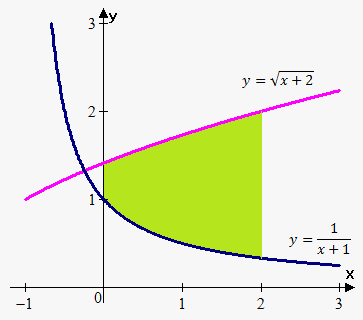Example 1. Find the area of the region bounded above by ${y}=\sqrt{{{x}+{2}}}$, bounded below by ${y}=\frac{{1}}{{{x}+{1}}}$, and bounded on the sides by ${x}={0}$ and ${x}={2}$.

The upper boundary curve is ${f{{\left({x}\right)}}}=\sqrt{{{x}+{2}}}$ and the lower boundary curve is ${y}=\frac{{1}}{{{x}+{1}}}$. Therefore, ${A}={\int_{{0}}^{{2}}}{\left(\sqrt{{{x}+{2}}}-\frac{{1}}{{{x}+{1}}}\right)}{d}{x}={\left(\frac{{2}}{{3}}{{\left({x}+{2}\right)}}^{{\frac{{3}}{{2}}}}-{\ln{{\left({x}+{1}\right)}}}\right)}{{\mid}_{{0}}^{{2}}}=$

$={\left(\frac{{2}}{{3}}\cdot{8}-{\ln{{\left({3}\right)}}}\right)}-{\left(\frac{{2}}{{3}}{{2}}^{{\frac{{3}}{{2}}}}-{\ln{{\left({1}\right)}}}\right)}=\frac{{16}}{{3}}-\frac{{4}}{{3}}\sqrt{{{8}}}-{\ln{{\left({3}\right)}}}$.

Now let's see how to handle situations when we are not given limits for integration.

Example 2. Find the area of the region enclosed by the parabola ${y}={5}{x}-{{x}}^{{2}}$ and line ${y}={x}$.

We are not given points bounds of integration here, so we need to find them.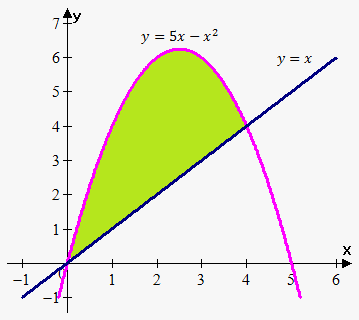For this need to find points of intersections of curves. To do this we must solve system

${\left\{\begin{array}{c}{y}={5}{x}-{{x}}^{{2}}\\{y}={x}\\ \end{array}\right.}$.

From this ${5}{x}-{{x}}^{{2}}={x}$ or ${x}{\left({x}-{4}\right)}={0}$. Solutions are ${x}={0}$ and ${x}={4}$. Thus, points of intersection are (0,0) and (4,4)

Top boundary curve is ${y}={5}{x}-{{x}}^{{2}}$ and lower boundary curve is ${y}={x}$. Therefore, ${A}={\int_{{0}}^{{4}}}{\left({\left({5}{x}-{{x}}^{{2}}\right)}-{x}\right)}{d}{x}={\int_{{0}}^{{4}}}{\left({4}{x}-{{x}}^{{2}}\right)}{d}{x}={\left({2}{{x}}^{{2}}-\frac{{1}}{{3}}{{x}}^{{3}}\right)}{{\mid}_{{0}}^{{4}}}=$

$={\left({2}\cdot{{4}}^{{2}}-\frac{{1}}{{3}}\cdot{{4}}^{{3}}\right)}={32}-\frac{{64}}{{3}}=\frac{{32}}{{3}}$.

Now, let's see in what situations we need to split interval of integration into parts.

Example 3. Find the area of the region enclosed by ${y}={5}{x}-{{x}}^{{2}}$ and ${y}={x}$ on interval ${\left[-{1},{5}\right]}$.

This example is extension of example 2. We encounter here problem. Function ${f{{\left({x}\right)}}}={5}{x}-{{x}}^{{2}}$ is not greater than ${g{{\left({x}\right)}}}={x}$ on interval ${\left[-{1},{5}\right]}$.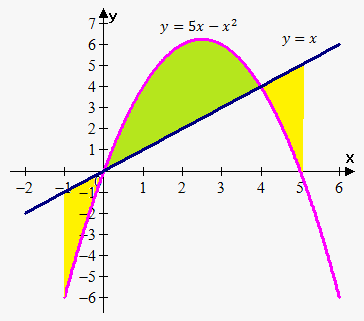To solve this problem we must divide interval ${\left[-{1},{5}\right]}$ on subintervals where ${f{{\left({x}\right)}}}\ge{g{{\left({x}\right)}}}$ and where ${g{{\left({x}\right)}}}\ge{f{{\left({x}\right)}}}$.

First subinterval is ${\left[-{1},{0}\right]}$: here ${f{{\left({x}\right)}}}\le{g{{\left({x}\right)}}}$ so ${A}_{{1}}={\int_{{-{1}}}^{{0}}}{\left({x}-{\left({5}{x}-{{x}}^{{2}}\right)}\right)}{d}{x}=$

$={\int_{{-{1}}}^{{0}}}{\left({{x}}^{{2}}-{4}{x}\right)}{d}{x}={\left(\frac{{1}}{{3}}{{x}}^{{3}}-{2}{{x}}^{{2}}\right)}{{\mid}_{{-{1}}}^{{0}}}=-{\left(-\frac{{1}}{{3}}-{2}\right)}=\frac{{7}}{{3}}$.

Second subinterval is ${\left[{0},{4}\right]}$: here ${f{{\left({x}\right)}}}\ge{g{{\left({x}\right)}}}$ and we found area of this region in example 2, so ${A}_{{2}}=\frac{{32}}{{3}}$.

Third subinterval is ${\left[{4},{5}\right]}$: here again ${f{{\left({x}\right)}}}\ge{g{{\left({x}\right)}}}$ so ${A}_{{1}}={\int_{{4}}^{{5}}}{\left({x}-{\left({5}{x}-{{x}}^{{2}}\right)}\right)}{d}{x}={\int_{{4}}^{{5}}}{\left({{x}}^{{2}}-{4}{x}\right)}{d}{x}={\left(\frac{{1}}{{3}}{{x}}^{{3}}-{2}{{x}}^{{2}}\right)}{{\mid}_{{4}}^{{5}}}=$

$={\left(\frac{{1}}{{3}}\cdot{{5}}^{{3}}-{2}\cdot{{5}}^{{2}}\right)}-{\left(\frac{{1}}{{3}}\cdot{{4}}^{{3}}-{2}\cdot{{4}}^{{2}}\right)}=\frac{{7}}{{3}}$.

Finally total area is ${A}=\frac{{7}}{{3}}+\frac{{32}}{{3}}+\frac{{7}}{{3}}=\frac{{46}}{{3}}$.

Following example shows that sometimes it is better to treat function as function of ${y}$ rather than ${x}$.

Example 4. Find the area enclosed by the line ${y}={x}+{1}$ and parabola ${{y}}^{{2}}={2}{x}+{5}$.

Here the first problem is that on different intervals we have different lower bounds. The other problem that we need express ${y}$ from ${{y}}^{{2}}={2}{x}+{6}$. We obtain 2 curves: ${y}=\pm\sqrt{{{2}{x}+{5}}}$. This adds work to find area.

So, it is better to use formula when ${x}$ is a function of ${y}$.

But for educational purposes let's do it in two ways.

First find points of intersection:

${\left\{\begin{array}{c}{y}={x}+{1}\\{{y}}^{{2}}={2}{x}+{5}\\ \end{array}\right.}$

Or ${{\left({x}+{1}\right)}}^{{2}}={2}{x}+{5}$. From this we have that ${{x}}^{{2}}={4}$. Solving this equation we obtain that ${x}=\pm{2}$.

Thus, points of intersection are (-2,-1) and (2,3).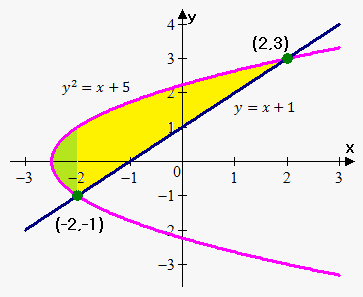Also, point where ${y}={0}$ for curve ${{y}}^{{2}}={2}{x}+{5}$ is ${x}=-\frac{{5}}{{2}}$.

Let's first solve regarding ${y}$ as a function of ${x}$. This will show the two problems we talked about.

First problem is that ${{y}}^{{2}}={2}{x}+{5}$ or ${y}=\pm\sqrt{{{2}{x}+{5}}}$. This gives two curves.

Second problem is that we can't integrate directly. We must divide required area into two areas: green area and yellow area, because there are different lower boundary curves:

For green area lower boundary curve is $-\sqrt{{{2}{x}+{5}}}$, so ${A}_{{1}}={\int_{{-\frac{{5}}{{2}}}}^{{-{2}}}}{\left(\sqrt{{{2}{x}+{5}}}-{\left(-\sqrt{{{2}{x}+{5}}}\right)}\right)}{d}{x}={2}{\int_{{-\frac{{5}}{{2}}}}^{{-{2}}}}\sqrt{{{2}{x}+{5}}}{d}{x}=\frac{{2}}{{3}}{{\left({2}{x}+{5}\right)}}^{{\frac{{3}}{{2}}}}{{\mid}_{{-\frac{{5}}{{2}}}}^{{-{2}}}}=$

$=\frac{{2}}{{3}}\cdot{1}=\frac{{2}}{{3}}$.

For yellow area lower boundary curve is ${y}={x}+{1}$, so ${A}_{{2}}={\int_{{-{2}}}^{{{2}}}}{\left(\sqrt{{{2}{x}+{5}}}-{\left({x}+{1}\right)}\right)}{d}{x}={\int_{{-{2}}}^{{2}}}{\left(\sqrt{{{2}{x}+{5}}}-{x}-{1}\right)}{d}{x}={\left(\frac{{1}}{{3}}{{\left({2}{x}+{5}\right)}}^{{\frac{{3}}{{2}}}}-\frac{{1}}{{2}}{{x}}^{{2}}-{x}\right)}{{\mid}_{{-{2}}}^{{2}}}=$

$={9}-{2}-{2}-{\left(\frac{{1}}{{3}}-{2}+{2}\right)}=\frac{{14}}{{3}}$.

Therefore, ${A}={A}_{{1}}+{A}_{{2}}=\frac{{2}}{{3}}+\frac{{14}}{{3}}=\frac{{16}}{{3}}$.

If we now treat ${x}$ as function of ${y}$ then equations of curves are: ${x}={y}-{1}$ and ${x}=\frac{{1}}{{2}}{\left({{y}}^{{2}}-{5}\right)}$, bounds for ${y}$ are from -1 to 3 so${A}={\int_{{-{1}}}^{{3}}}{\left({y}-{1}-\frac{{1}}{{2}}{\left({{y}}^{{2}}-{5}\right)}\right)}{d}{y}={\left(\frac{{1}}{{2}}{{y}}^{{2}}-\frac{{1}}{{6}}{{y}}^{{3}}+\frac{{3}}{{2}}{y}\right)}{{\mid}_{{-{1}}}^{{3}}}=\frac{{9}}{{2}}-\frac{{9}}{{2}}+\frac{{9}}{{2}}-{\left(\frac{{1}}{{2}}+\frac{{1}}{{6}}-\frac{{3}}{{2}}\right)}=\frac{{16}}{{3}}$.

As can be seen second method is much easier.